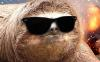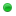Everyone who has never used our Bots before, can test each one for 2 days without any limitation.
The trial is given automatically when you login on the Bot, but in some cases it wouldn't work (security reasons).
If this happens, send me a private message and i will be checking the failed trials manually and adding it for those who didn't get it.
We are looking for resellers who may accept payment methods different from ours, including classictibia's cash, realesta's cash, mastercores' cash, etc. Interested? Click here at anytime.

 Use rune to remove itemsp4tr1kFree User Posts: 3 Threads: 2 Joined: Jan 2022 Reputation: 0 02-04-2022, 08:14 PM Okay I have been sitting with this all day but i cant figure out how tables work so i need help, big time |Only Registered members can see download links. | Click here to buy subscription or here to register.|Only Registered members can see download links. | Click here to buy subscription or here to register.alt="Sad" title="Sad" class="smilie smilie_8" /> truly sorry to bother you again but i have nowere to turn! What i need is a script that checks the sqms around him, except 2(east and north) If he finds random item, dosnt matter which. (NE, SE, S, SW, W, NW) he uses desinegrate rune on 1x of these sqms (at random)  Just to be clear, he dosnt have to clear all of them, just do 1 use of the rune and move on. what i got so far so you might get an idea of what i need. (COPY)```if gettile(32339, 32850, 7).itemcount > 1 then Problem1=true else Problem1=false end Solution1=useitemon(3197, 32339, 32850, 7) if gettile(32339, 32852, 7).itemcount > 1 then Problem2=true else Problem2=false end Solution2=useitemon(3197, 32339, 32852, 7) if gettile(32338, 32852, 7).itemcount > 1 then Problem3=true else Problem3=false end Solution3=useitemon(3197, 32338, 32852, 7) if gettile(32337, 32852, 7).itemcount > 1 then Problem4=true else Problem4=false end Solution4=useitemon(3197, 32337, 32852, 7) if gettile(32337, 32851, 7).itemcount > 1 then Problem5=true else Problem5=false end Solution5=useitemon(3197, 32337, 32851, 7) if gettile(32337, 32850, 7).itemcount > 1 then Problem6=true else Problem6=false end Solution6=useitemon(3197, 32337, 32850, 7) if Problem1==true or Problem2==true or Problem3==true or Problem4==true or Problem5==true or Problem6==true then math.random(Solution1, Solution2, Solution3, Solution4, Solution5, Solution6) end```ArkilysAdministratorPosts: 2,532 Threads: 422 Joined: Jul 2018 Reputation: 68 02-05-2022, 02:35 AM Hello. I didn't tested your code, it seems to work fine. Except the last line (math.random...). Try something like this. (COPY)```local LOCATIONS = { -- Locations to check for items.   { X = 12341, Y = 12345, Z = 6 },   { X = 12342, Y = 12345, Z = 6 },   { X = 12343, Y = 12345, Z = 6 },   { X = 12344, Y = 12345, Z = 6 },   { X = 12345, Y = 12345, Z = 6 }, } if tableShuffle == nil then   function tableShuffle(table)     local index     for i = #table, 1, -1 do         index = _random(1, i)         table[i], table[index] = table[index], table[i]     end     return table   end end LOCAITONS = tableShuffle(LOCATIONS) for _, location in ipairs(LOCATIONS) do   local tile = gettile(location.X, location.Y, location.Z)   if tile.itemcount > 1 then       local isMoveable = true       local isPickupable = false       local flags = itemflags(tile.topitem.id)       for __, flag in ipairs(flags) do           if flag == 13 then               isMoveable = false           elseif flag == 16 then               isPickupable = true               break           end       end       if isMoveable or isPickupable then           useitemon(3197, location.X, location.Y, location.Z)           wait(500, 800)       end   end end```